Anúncio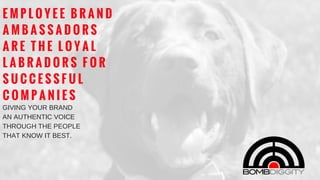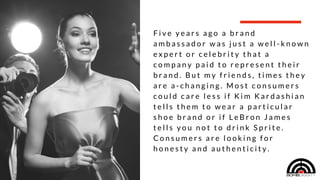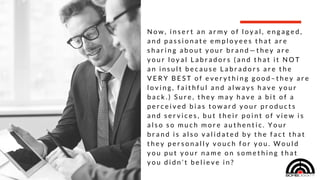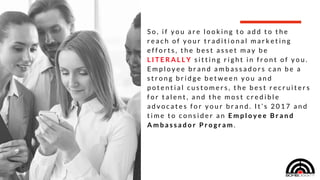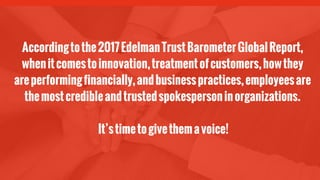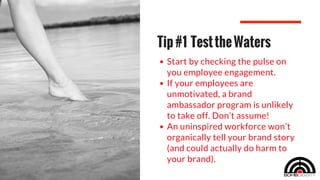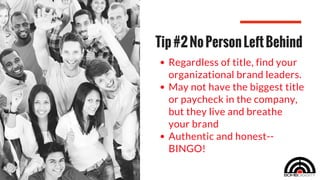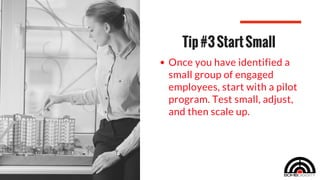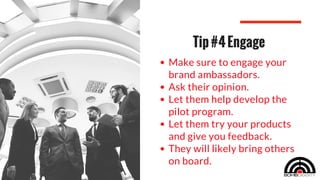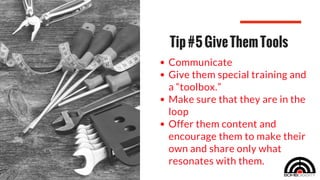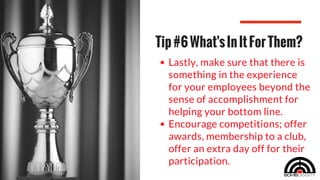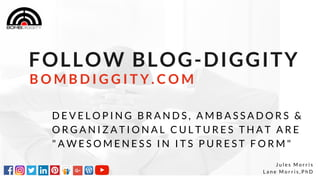1 de 13
Anúncio

### EMPLOYEE BRAND AMBASSADORS ARE THE LOYAL LABRADORS FOR SUCCESSFUL COMPANIES—GIVING YOUR BRAND AN AUTHENTIC VOICE THROUGH THE PEOPLE THAT KNOW IT BEST.

1. E M P L O Y E E B R A N D A M B A S S A D O R S A R E T H E L O Y A L L A B R A D O R S F O R S U C C E S S F U L C O M P A N I E S GIVING YOUR BRAND AN AUTHENTIC VOICE THROUGH THE PEOPLE THAT KNOW IT BEST.
2. F i v e y e a r s a g o a b r a n d a m b a s s a d o r w a s j u s t a w e l l - k n o w n e x p e r t o r c e l e b r i t y t h a t a c o m p a n y p a i d t o r e p r e s e n t t h e i r b r a n d . B u t m y f r i e n d s , t i m e s t h e y a r e a - c h a n g i n g . M o s t c o n s u m e r s c o u l d c a r e l e s s i f K i m K a r d a s h i a n t e l l s t h e m t o w e a r a p a r t i c u l a r s h o e b r a n d o r i f L e B r o n J a m e s t e l l s y o u n o t t o d r i n k S p r i t e . C o n s u m e r s a r e l o o k i n g f o r h o n e s t y a n d a u t h e n t i c i t y .
3. W h e n w a s t h e l a s t t i m e t h a t w o r d - o f - m o u t h i n f l u e n c e d a p u r c h a s e o r d e c i s i o n t h a t y o u m a d e ? M a y b e a g o o d f r i e n d t o l d y o u a b o u t a g r e a t a c c o u n t a n t t h a t t h e y u s e d o r a n a w e s o m e r e s t a u r a n t … M a y b e a c o - w o r k e r g a v e y o u a c o d e f o r a f r e e R e d B o x r e n t a l o r t h e n a m e o f a c o n t r a c t o r t h a t t h e y r e a l l y l i k e d … W h e r e v e r i t c a m e f r o m , y o u l i k e l y a c t e d o n i t b e c a u s e y o u T R U S T E D t h e m . T h i s i s w h e r e m a r k e t i n g i s i n 2 0 1 7 — a n d d o e s n ’ t i t j u s t m a k e m o r e s e n s e ?
4. N o w , i n s e r t a n a r m y o f l o y a l , e n g a g e d , a n d p a s s i o n a t e e m p l o y e e s t h a t a r e s h a r i n g a b o u t y o u r b r a n d — t h e y a r e y o u r l o y a l L a b r a d o r s ( a n d t h a t i t N O T a n i n s u l t b e c a u s e L a b r a d o r s a r e t h e V E R Y B E S T o f e v e r y t h i n g g o o d – t h e y a r e l o v i n g , f a i t h f u l a n d a l w a y s h a v e y o u r b a c k . ) S u r e , t h e y m a y h a v e a b i t o f a p e r c e i v e d b i a s t o w a r d y o u r p r o d u c t s a n d s e r v i c e s , b u t t h e i r p o i n t o f v i e w i s a l s o s o m u c h m o r e a u t h e n t i c . Y o u r b r a n d i s a l s o v a l i d a t e d b y t h e f a c t t h a t t h e y p e r s o n a l l y v o u c h f o r y o u . W o u l d y o u p u t y o u r n a m e o n s o m e t h i n g t h a t y o u d i d n ’ t b e l i e v e i n ?
5. S o , i f y o u a r e l o o k i n g t o a d d t o t h e r e a c h o f y o u r t r a d i t i o n a l m a r k e t i n g e f f o r t s , t h e b e s t a s s e t m a y b e L I T E R A L L Y s i t t i n g r i g h t i n f r o n t o f y o u . E m p l o y e e b r a n d a m b a s s a d o r s c a n b e a s t r o n g b r i d g e b e t w e e n y o u a n d p o t e n t i a l c u s t o m e r s , t h e b e s t r e c r u i t e r s f o r t a l e n t , a n d t h e m o s t c r e d i b l e a d v o c a t e s f o r y o u r b r a n d . I t ’ s 2 0 1 7 a n d t i m e t o c o n s i d e r a n E m p l o y e e B r a n d A m b a s s a d o r P r o g r a m .
6. Accordingtothe2017EdelmanTrustBarometerGlobalReport, whenitcomestoinnovation,treatmentofcustomers,howthey areperformingfinancially,andbusinesspractices,employeesare themostcredibleandtrustedspokespersoninorganizations. It’stimetogivethemavoice!
7. Start by checking the pulse on you employee engagement.  If your employees are unmotivated, a brand ambassador program is unlikely to take off. Don’t assume!  An uninspired workforce won’t organically tell your brand story (and could actually do harm to your brand).  Tip#1 TesttheWaters
8. Regardless of title, find your organizational brand leaders. May not have the biggest title or paycheck in the company, but they live and breathe your brand Authentic and honest-- BINGO! Tip#2NoPersonLeftBehind
9. Once you have identified a small group of engaged employees, start with a pilot program. Test small, adjust, and then scale up. Tip#3StartSmall
10. Make sure to engage your brand ambassadors.  Ask their opinion.  Let them help develop the pilot program.  Let them try your products and give you feedback.  They will likely bring others on board. Tip#4Engage
11. Communicate  Give them special training and a “toolbox.”  Make sure that they are in the loop  Offer them content and encourage them to make their own and share only what resonates with them. Tip#5GiveThemTools
12. Lastly, make sure that there is something in the experience for your employees beyond the sense of accomplishment for helping your bottom line. Encourage competitions; offer awards, membership to a club, offer an extra day off for their participation. Tip#6What'sInItForThem?
13. FOLLOW BLOG-DIGGITY J u l e s M o r r i s L a n e M o r r i s , P h D B O M B D I G G I T Y . C O M D E V E L O P I N G B R A N D S , A M B A S S A D O R S & O R G A N I Z A T I O N A L C U L T U R E S T H A T A R E " A W E S O M E N E S S I N I T S P U R E S T F O R M "
Anúncio